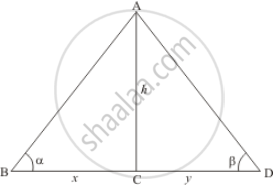# From the top of a lighthouse, the angles of depression of two ships on the opposite sides of it are observed to be a and 3. If the height of the lighthouse be h meters and the line joining the ships passes through the foot of the lighthouse, show that the distance - Mathematics

From the top of a lighthouse, the angles of depression of two ships on the opposite sides of it are observed to be a and 3. If the height of the lighthouse be h meters and the line joining the ships passes through the foot of the lighthouse, show that the distance

(h(tan alpha + tan beta))/(tan alpha tan beta) meters

#### Solution

Let h be the height of lighthouse AC. And an angle of depression of the top of the lighthouse from two ships is α and β respectively.

Let BC =  x, CD = y. And ∠ABC = α, ∠ADC = β.

We have to find the distance between the ships

We have the corresponding figure as followsWe use trigonometric ratios.

In ΔABC

=> tan α = (AC)/(BC)

=> tan α = h/x

=> tan β = (AC)/(CD)

=> tan β = h/y

=> y = h/(tan β)

Now

=> BD = x + y

=> BD = h/(tan α) + h/(tan β)

=> BD = (h(tan α + tan β))/(tan α tan β)

Hence the distance between ships is (h(tan α + tan β))/(tan α tan β)

Concept: Heights and Distances
Is there an error in this question or solution?
Chapter 12: Trigonometry - Exercise 12.1 [Page 34]

#### APPEARS IN

RD Sharma Class 10 Maths
Chapter 12 Trigonometry
Exercise 12.1 | Q 62 | Page 34

Share